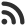Feed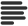Articles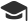Tutorials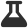Lab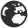CompaniesLeaderboard
 DG Student at BIT Mesra Aug. 26, 2020, 11:46 a.m. ⋅ 583 views

# Wipro Placement Aptitude Questions with answers (set 2)

Q1. 6 men,8 women and 6 children complete a job for a sum of Rs.950. If their individual wages are in the ratio 4:3:2,
the total money earned by the children is(in rupees)

A. 160
B. 190
C. 220
D. 170

Ans. B

Q2. There is an element which triplicates in every hour. Each of these 3 items inturn reproduce exactly 3 other items.
If a single compound is kept in a container at noon and the container is full by midnight. After how many hours is the
container 1/3 full?

A. 11:00am
B. 10:00pm
C. 11:00pm
D. 10:00am

Ans. C

Q3. A sum of Rs 468.75 was lent out at simple interest and at the end of 1 year and 8 months, the
total amount of Rs 500 is received. find the rate of interest.

A. 2%
B. 4%
C. 1%
D. 3%

Ans. B

Q4. A sum was put at simple interest at a certain rate for 3 years. Had it been put at 2% higher rate, it would have fetched Rs.360 more. Find the sum.

A. Rs.4000
B. Rs.9000
C. Rs.5000
D. Rs.6000

Ans. D

Q5. A person goes to a bank and Quotes x Rs and y paise on a cheque. The cashier misreads it and gives y Rs and x paise. The man comes out and donates 5 paise to a beggar. Now, the man has exactly double the amount he has quoted on the cheque.

A. 35 Rs. and 65 paise.
B. 32 Rs. and 63 paise.
C. 30 Rs. and 65 paise.
D. 31 Rs. and 63 paise.

Ans. D

Q6. A positive integer is selected at random and is divided by 7,what is the probability that the
remainder is 1?

A. 3/7
B. 4/7
C. 1/7
D. 2/7

Ans. C

Q7. The number of oranges in the three baskets are in the ratio of 3:4:5. In which ratio the number of oranges in first two
baskets must be increased so that the new ratio becomes 5:4:3?

A. 1:3
B. 2:1
C. 3:4
D. 2:3

Ans. B

Q8. A can do a piece of work in 100 days, B and C together can do the same work in 20 days. If B can do the work in same time as that of
C and A together then how long C alone can do the same work?

A. 100 days
B. 50 days
C. 25 days
D. 20 days

Ans. B

Q9. A mixture of 40 liters of salt and water contains 70% of salt.how much water must be added to
decrease the salt percentage to 40%?

A. 40 liters
B. 30 liters
C. 20 liters
D. 2 liters

Ans. B

Q10. If a man marks his goods 40% higher than the cost price and allows a discount of 20%, find his net gain.

A. 12%
B. 20%
C. 30
D. 28%

Ans. A

Q11. All of the students at a college are majoring in psychology, business, or both. 73% of the students are psychology majors,
& 62% are business majors. If there are 200 students, how many of them are majoring in both psychology & business?

A. 75
B. 80
C. 72
D. 70

Ans. D

Q12. If LCM of two number is 693, HCF of two numbers is 11 and one number is 99, then find
other.

A. 34
B. 77
C. 12
D. 45

Ans. B

Q13.On an escalator it took me 30 seconds and 26 steps to reach the bottom. However if I am able to step down 34 stairs
I would only require 18 seconds to go to bottom. How many steps were there in escalator when it was idle?

A. 20
B. 30
C. 46
D. indeterminable

Ans. C

Q14. Grass in lawn grows equally thick and in a uniform rate. It takes 24 days for 70 cows and 60 for 30 cows . How many cows can eat away the same in 96 days?

A. 18
B. 20
C. 21
D. 19

Ans. A

Q15. Find the greatest number which on dividing 1661 and 2045, leaves a remainder of 10 and
13 respectively

A. 125
B. 127
C. 129
D. 131

Ans. B

Q16. In a mixture of 63 liters, the milk and water are in the ratio 7:2. If 7 liters of water is added to the mixture, find the ratio in the resulting mixture.

A. 3:1
B. 4:1
C. 7:3
D. 7:5

Ans. C

Q17. There is a certain four digit number whose fourth digit is twice the first digit.Third digit is three more than second digit. Sum of the first and fourth digits twice the third number.What was that number ?

A. 2034
B. 4368
C. a and b
D. None of these

Ans. C

Q18. The greatest number which on dividing 1657 and 2037 leaves remainders 6 and 5 respectively, is:

A. 123
B. 127
C. 235
D. 305

Ans. B

Q19. A thief steals half the total no of loaves of bread plus 1/2 loaf from a bakery. A second thief steals half the remaining
no of loaves plus 1/2 loaf and so on. After the 5th thief has stolen there are no more loaves left in the bakery. What was
the total no of loaves did the bakery have at the beginning?

A. 35
B. 41
C. 28
D. 31

Ans. D

Q20. A and B can together finish a work 30 days. They worked together for 20 days and then B left. After another 20 days, A finished the remaining work. In how many days A alone can finish the work?

A. 40
B. 50
C. 54
D. 60

Ans. D

Q21. A person needs 6 steps to cover a distance of one slaB. if he increases his foot length(step length) by
3 inches he needs only 5 steps to cover the slabs length. what is the length of the each slab?

A. 31 inches
B. 24 inches
C. 26 inches
D. 43 inches

Ans. A

Q22. A garrison of 3300 men has provisions for 32 days, when given at a rate of 850 grams per heaD. At the end of 7 days a
reinforcement arrives and it was found that now the provisions will last 8 days less, when given at the rate of 825 grams
per heaD.How, many more men can it feed?

A. 1700 men
B. 1800 men
C. 2000 men
D. 2500 men

Ans. A

Q23. From 5 different green balls, four different blue balls and three different red balls, how many combinations of balls can
be chosen taking at least one green and one blue ball?

A. 3720
B. 3890
C. 3520
D. 3600

Ans. A

Q24. Three pipes, A, B, and C are attached to a tank. A and B can fill it in 20 and 30 minutes respectively while C can empty
it in 15 minutes.If A, B & C are kept open successively for 1 minute each, how soon will the tank be filled?

A. 167 minutes
B. 177 minutes
C. 188 minutes
D. 196 minutes

Ans. A

Q25. A person walking 5/6 of his usual rate is 40 minutes late. What is his usual time?

A. 6 hours 20 minutes
B. 4 hours 40 minutes.
C. 3 hours 30 minutes.
D. 3 hours 20 minutes.

Ans. D

Q26. The minute hand of a clock overtakes the hour hand at intervals of 64 minutes of correct time. How much a day does the clock gain or lose?

A. 36 8/11 minutes
B. 32 11/8 minutes
C. 32 8/11 minutes
D. 30 8/11 minutes

Ans. B

Q27. Solve for x and y: 1/x – 1/y = 1/3, 1/x2 + 1/y2 = 5/9.

A. x = 3/2 or -3 and y = 3 or -3/2.
B. x = 2/3 or -3 and y = 2 or -3/2.
C. x = 4/2 or -3 and y = 2 or -3/2.
D. x = 3/2 or -3 and y = 3 or -2/3.

Ans. A

Q28. Daal is now being sold at Rs. 20 a kg. During last month its rate was Rs. 16 per kg. By how much percent should a family reduce its
consumption so as to keep the expenditure fixed?

A. 30 %.
B. 20 %.
C. 35 %.
D. 40 %.

Ans. B

Q29. Find the sum of the first 14 terms for a sequence starting with 2, ending with 120 and common difference 2.

A. 845
B. 854
C. 800
D. 860

Ans. B

Q30. Find the difference between last and last but one term in the Sequence 1, 9, 17, 25… which has 40 terms in total…

A. 8
B. 16
C. 24
D. 12

Ans. A

Q31. Find the 7th term in the series 4,8,16…

A. 512
B. 256
C. 64
D. 200

Ans. B

Q32. Find the sum of the first 5 terms in 3,9,27

A. 363
B. 121
C. 242
D. 352

Ans. A

Events

Nov. 28, 2018, 5:30 p.m.

Python from zero to hero

place Delhi

Aug. 13, 2018, 5:30 p.m.

Python from zero to hero

place Bangalore ( HackersFriend office BTM Layout)